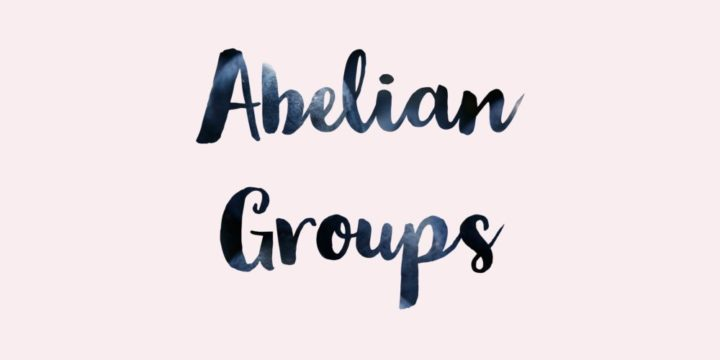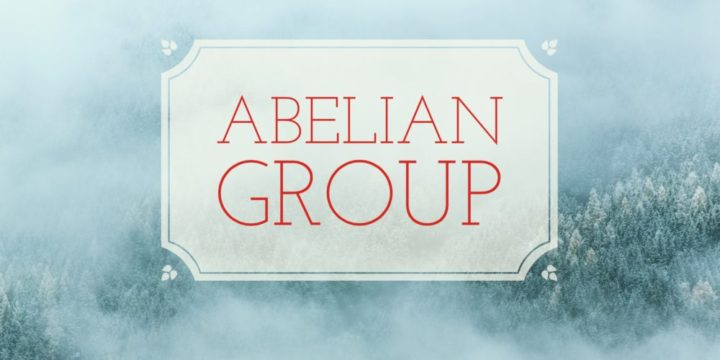# Torsion Subgroup of an Abelian Group, Quotient is a Torsion-Free Abelian Group## Problem 307

Let $A$ be an abelian group and let $T(A)$ denote the set of elements of $A$ that have finite order.

(a) Prove that $T(A)$ is a subgroup of $A$.

(The subgroup $T(A)$ is called the torsion subgroup of the abelian group $A$ and elements of $T(A)$ are called torsion elements.)

(b) Prove that the quotient group $G=A/T(A)$ is a torsion-free abelian group. That is, the only element of $G$ that has finite order is the identity element.Add to solve later

## Proof.

### (a) $T(A)$ is a subgroup of $A$

We write the group operation multiplicatively.
Let $x, y\in T(A)$. Then $x, y$ have finite order, hence there exists positive integers $m, n$ such that $x^m=e, y^n=e$, where $e$ is the identity element of $A$. Then we have
\begin{align*}
(xy)^{mn}&=x^{mn}y^{mn} \qquad \text{ (since $A$ is abelian)}\\
&=(x^m)^n(y^m)^n=e^me^n=e.
\end{align*}
Therefore the element $xy$ has also finite order, hence $xy \in T(A)$.

Also, we have
\begin{align*}
(x^{-1})^m=(x^m)^{-1}=e^{-1}=e.
\end{align*}
Hence the inverse $x^{-1}$ of $x$ has finite order, hence $x^{-1}\in T(A)$.

Therefore, the subset $T(A)$ is closed under group operation and inverse, hence $T(A)$ is a subgroup of $A$.

### (b) $A/T(A)$ is a torsion-free abelian group

Since $A$ is an abelian group, the quotient $G=A/T(A)$ is also an abelian group.
For $a\in A$, let $\bar{a}=aT(A)$ be an element of $G=A/T(A)$. Suppose that $\bar{a}$ has finite order in $G$. We want to prove that $\bar{a}=\bar{e}$ the identity element of $G$.

Since $\bar{a}$ has finite order, there exists a positive integer $n$ such that
$\bar{a}^n=\bar{e}.$ This implies that
$a^nT(A)=T(A)$ and thus $a^n\in T(A)$.

Since each element of $T(A)$ has finite order by definition, there exists a positive integer $m$ such that $(a^n)^m=e$.
It follows from $a^{nm}=e$ that $a$ has finite order, and thus $a\in T(A)$.
Therefore we have
$\bar{a}=aT(A)=T(A)=\bar{e}.$

We have proved that any element of $G=A/T(A)$ that has finite order is the identity, hence $G$ is the torsion-free abelian subgroup of $G$.Add to solve later

### More from my site

#### You may also like...

This site uses Akismet to reduce spam. Learn how your comment data is processed.

###### More in Group Theory##### If a Group $G$ Satisfies $abc=cba$ then $G$ is an Abelian Group

Let $G$ be a group with identity element $e$. Suppose that for any non identity elements $a, b, c$ of...

Close